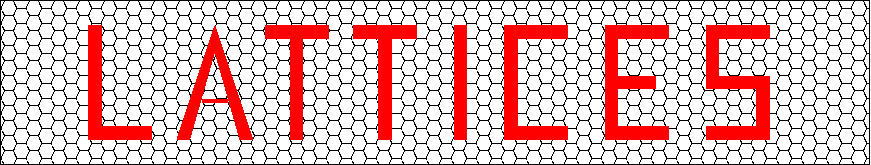## Lattices obtained from the extremal unimodular 72 dimensional lattice

An entry from the Catalogue of Lattices, which is a joint project of
Gabriele Nebe, RWTH Aachen University (nebe@math.rwth-aachen.de) and
Neil J. A. Sloane, AT&T Shannon Labs (njasloane@gmail.com)## Contents of this file• The Hermitian structure of Leech over sqrt(-7) used to construct the extremal 72 dimensional unimodular lattice Gamma commutes with a suitable structure of Leech over sqrt(5).
Let F be some Gram matrix of the Leech lattice and F5=(5+\sqrt{5})/2 F. Then the lattice with Gram matrix F5 has minimum norm 8 and hence is an extremal 5-modular lattice of dimension 24. A short computer calculation shows that the Turyn construction applied to F5 yields a 5-modular lattice of minimum 16. Note that this is the same lattice as Gamma, we just changed the quadratic form from F^3 to F5^3. The lattices Gamma with respect to (nF^3+F5^3) hence define modular lattices of level (n^2+5n+5) and minimum 16+8n (n=0,1,2, ...). In the limit the density of these lattices tends to the density of the extremal even unimodular lattice. A computation with Hilbert modular forms shows that the Hilbert Theta Series of the lattice Gamma over Z[(1+sqrt(5))/2] is uniquely determined by the fact that the unimodular lattice (Gamma,F^3) has minimum 8. In particular all 75411000 minimal vectors of (Gamma,F5^3) are also minimal vectors of (Gamma,F^3), so the kissing number of all lattices (Gamma, nF^3+F5^3) is 75411000 (whereas the one of (Gamma,F^3) is 6218175600.

• LAST_LINE

Haftungsausschluss/Disclaimer

Gabriele Nebe